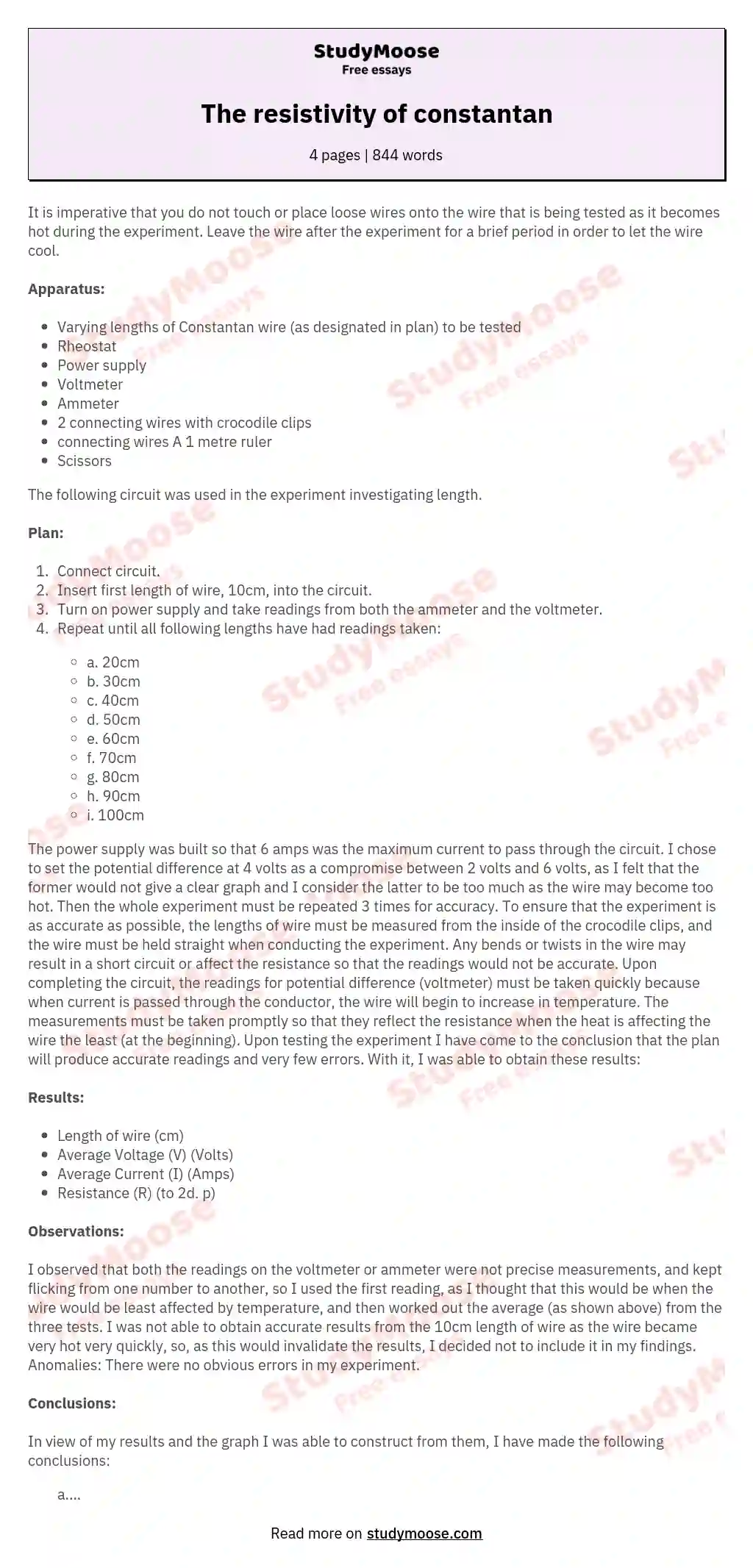# The resistivity of constantan

It is imperative that you do not touch or place loose wires onto the wire that is being tested as it becomes hot during the experiment. Leave the wire after the experiment for a brief period in order to let the wire cool.

#### Apparatus:

• Varying lengths of Constantan wire (as designated in plan) to be tested
• Rheostat
• Power supply
• Voltmeter
• Ammeter
• 2 connecting wires with crocodile clips
• connecting wires A 1 metre ruler
• Scissors

The following circuit was used in the experiment investigating length.

#### Plan:

1. Connect circuit.
2. Insert first length of wire, 10cm, into the circuit.
3. Turn on power supply and take readings from both the ammeter and the voltmeter.
• a. 20cm
• b. 30cm
• c. 40cm
• d. 50cm
• e. 60cm
• f. 70cm
• g. 80cm
• h. 90cm
• i. 100cm

The power supply was built so that 6 amps was the maximum current to pass through the circuit. I chose to set the potential difference at 4 volts as a compromise between 2 volts and 6 volts, as I felt that the former would not give a clear graph and I consider the latter to be too much as the wire may become too hot.

Get quality help nowWriter LylaVerified writer

Proficient in: Electricity5 (876)

“ Have been using her for a while and please believe when I tell you, she never fail. Thanks Writer Lyla you are indeed awesome ”+84 relevant experts are online

Then the whole experiment must be repeated 3 times for accuracy. To ensure that the experiment is as accurate as possible, the lengths of wire must be measured from the inside of the crocodile clips, and the wire must be held straight when conducting the experiment. Any bends or twists in the wire may result in a short circuit or affect the resistance so that the readings would not be accurate.

Get to Know The Price Estimate For Your Paper
Topic
Number of pages
Email Invalid email

You won’t be charged yet!

Upon completing the circuit, the readings for potential difference (voltmeter) must be taken quickly because when current is passed through the conductor, the wire will begin to increase in temperature.

The measurements must be taken promptly so that they reflect the resistance when the heat is affecting the wire the least (at the beginning). Upon testing the experiment I have come to the conclusion that the plan will produce accurate readings and very few errors. With it, I was able to obtain these results:

#### Results:

• Length of wire (cm)
• Average Voltage (V) (Volts)
• Average Current (I) (Amps)
• Resistance (R) (to 2d. p)

#### Observations:

I observed that both the readings on the voltmeter or ammeter were not precise measurements, and kept flicking from one number to another, so I used the first reading, as I thought that this would be when the wire would be least affected by temperature, and then worked out the average (as shown above) from the three tests. I was not able to obtain accurate results from the 10cm length of wire as the wire became very hot very quickly, so, as this would invalidate the results, I decided not to include it in my findings. Anomalies: There were no obvious errors in my experiment.

#### Conclusions:

In view of my results and the graph I was able to construct from them, I have made the following conclusions:

a. As predicted, when the length of the wire increased, the resistance also increased.

b. The graph shows a strong trend forming a straight line, showing that the length is directly proportional to the resistance; as the length doubles the resistance doubles as well.

From my analysis, I can confidently say that my prediction was correct. From studying the manner in which electricity is conducted, one can explain why the length of a wire is directly proportional to the resistance.

The number of freely mobile electrons in a given length of wire will produce a specific amount of resistance. This is because as a current is passed through the conductor the electrons in the outer shells of the particles are given energy so that they can move. As they travel through the wire, they come into contact with impurities in the conductor, other particles and any immobile material with which they ‘bump’ into. This collision releases some of the electrical energy as heat energy, which is then lost to the surroundings.

When the given length is doubled, this means that there is double the amount of mobile electrons, and so double the chance of a collision. As an electrical current is passed through the conductor, an electron has to travel double the distance, and will therefore have two times the amount of objects in its path. Because there is twice the amount of collisions, there will also be twice the amount of electrical energy being converted into heat, and so the resistance doubles. From these results we can work out the resistivity of Constantan wire:

R = ρ l /A

There were very few mistakes in my experiment, as is shown by the straight line on the graph, but I have several things to consider while evaluating my experiment. Although my circuit included a rheostat to control the current, the current through the wire varied dramatically, and this will affect the temperature of the wire. If the readings for current and voltage were taken immediately after the circuit was connected, these fluctuating currents should not have interfered with the results.